Learning Library
premium

# 3.OA.D.9 Worksheets, Workbooks, Lesson Plans, and Games

#### CCSS.MATH.CONTENT.3.OA.D.9

:
"Identify arithmetic patterns (including patterns in the addition table or multiplication table), and explain them using properties of operations."

These worksheets, games, and lesson plans can help students practice this Common Core State Standards skill.

## Worksheets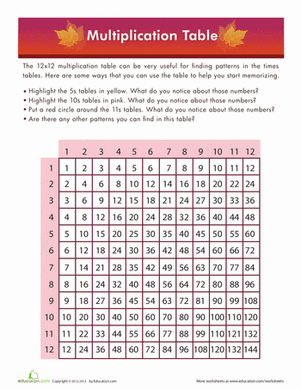Multiplication Times Table
Worksheet
Multiplication Times Table
Introduce your math whiz to times tables with this printable multiplication table. Follow the prompts to find some common patterns in the table.
3rd grade
Math
Worksheet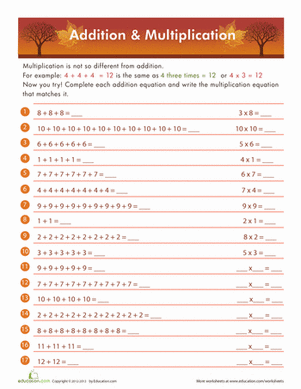Addition and Multiplication
Worksheet
Addition and Multiplication
Here's a helpful way to teach multiplication to a beginner. Show your child how an addition problem is similar to a multiplication problem.
3rd grade
Math
Worksheet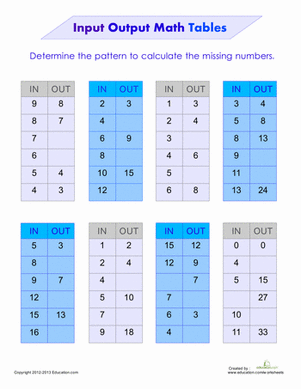Function Tables: Input Output
Worksheet
Function Tables: Input Output
Find the rule that links the input and output numbers in this chart! It could be addition, subtraction or multiplication; it's up to you to find the connection.
3rd grade
Math
Worksheet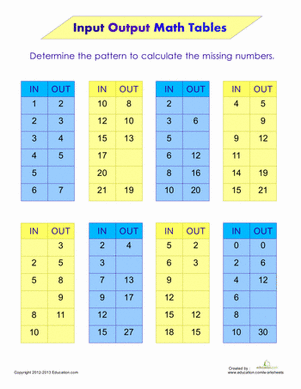Input Output Math Tables
Worksheet
Input Output Math Tables
Find the hidden rule in these puzzling math tables! Compare your "in" number to the corresponding "out" number to find the pattern in each table.
3rd grade
Math
Worksheet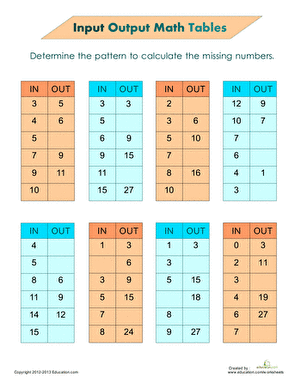Function Tables
Worksheet
Function Tables
Compare your "input" number to the corresponding "output" number to find the pattern in each table and fill in the missing numbers.
3rd grade
Math
Worksheet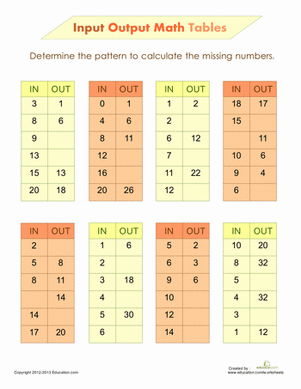Input Output Math
Worksheet
Input Output Math
Use a little bit of logic to find out the rule that links "input" and "output" in these math puzzles.
3rd grade
Math
Worksheet

## Lesson PlansBoom, Clap! Patterns in the Multiplication Table
Lesson plan
Boom, Clap! Patterns in the Multiplication Table
BOOM! Have a blast teaching your students how to find and use patterns within the multiplication table to increase fluency with multiplication and build number sense.
3rd grade
Math
Lesson plan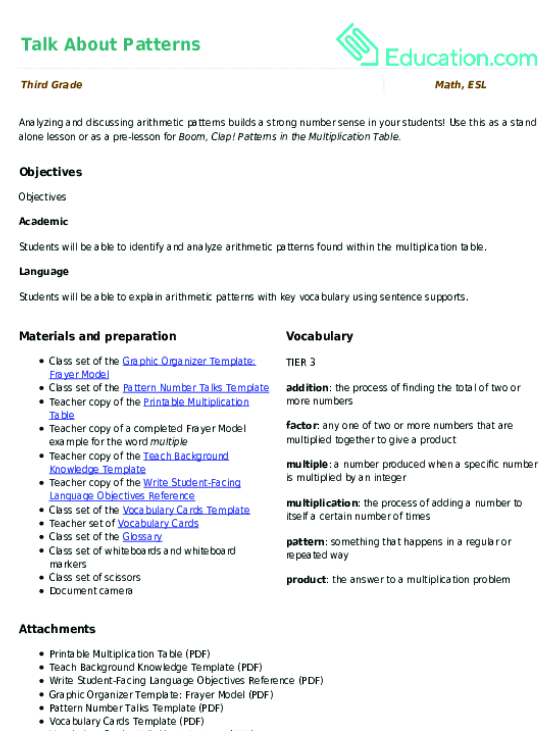Talk About Patterns
Lesson plan
Talk About Patterns
Analyzing and discussing arithmetic patterns builds a strong number sense in your students! Use this as a stand alone lesson or as a pre-lesson for *Boom, Clap! Patterns in the Multiplication Table.*
3rd grade
Math
Lesson plan

## Workbooks

No workbooks found for this common core node.

## GamesSir Whiskers Division Story
Story
Sir Whiskers Division Story
Kids learn all about division vocabulary and strategies with this silly story about a cat crew.
3rd grade
Math
Story

## Exercises

No exercises found for this common core node.

### Add to collection

Create new collection

0

### New Collection>

0 items

What could we do to improve Education.com?

Please note: Use the Contact Us link at the bottom of our website for account-specific questions or issues.

What would make you love Education.com?